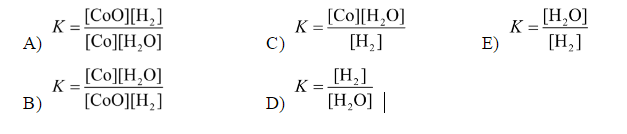# Problem: For the reaction,CoO(s) + H 2(g) ⇌ Co(s) + H 2O(g)at 550°C, the value of K is 67.  The equilibrium constant expression is

###### FREE Expert Solution
100% (67 ratings)
###### Problem Details

For the reaction,

CoO(s) + H 2(g) ⇌ Co(s) + H 2O(g)

at 550°C, the value of K is 67.  The equilibrium constant expression isWhat scientific concept do you need to know in order to solve this problem?

Our tutors have indicated that to solve this problem you will need to apply the Equilibrium Expressions concept. If you need more Equilibrium Expressions practice, you can also practice Equilibrium Expressions practice problems.

What is the difficulty of this problem?

Our tutors rated the difficulty ofFor the reaction,CoO(s) + H 2(g) ⇌ Co(s) + H 2O(g)at 550°C, ...as medium difficulty.

How long does this problem take to solve?

Our expert Chemistry tutor, Sabrina took 2 minutes and 1 second to solve this problem. You can follow their steps in the video explanation above.

What professor is this problem relevant for?

Based on our data, we think this problem is relevant for Professor Ramanoudjame's class at HCC.